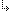- 4.45.2.60. -4. Standard Units4.45. Math - Extended Mathematics unit4.45.2. Math Unit Procedures and Functions4.45.2.60. Syd function

## 4.45.2.60. Syd function

Targets: MS-DOS, OS/2, Win32

Math Unit

Returns the sum of the year digits depreciation.

Declaration:
```function Syd(InitialValue,
Residue: Extended;
Period,
Time: DWord): Extended;```
Remarks:

The Syd function calculates depreciation amounts for an asset using an accelerated depreciation method. This allows for higher depreciation in the earlier years of an asset's life. InitialValue is the initial cost of the asset. Residue is the value of the asset at the end of its life expectancy. Time is the length of the asset's life expectancy. Period is the period for which to calculate the depreciation.

- 4.45.2.60. -